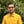Trusted answers to developer questions
Trusted Answers to Developer Questions

Related Tags

perl
sine
communitycreator

# What is sin() in Perl?Harris Amjad

Grokking Modern System Design Interview for Engineers & Managers

Ace your System Design Interview and take your career to the next level. Learn to handle the design of applications like Netflix, Quora, Facebook, Uber, and many more in a 45-min interview. Learn the RESHADED framework for architecting web-scale applications by determining requirements, constraints, and assumptions before diving into a step-by-step design process.

The sin() function returns the sine of a number. To be more specific, sin() returns the sine of a number in radians.

The illustration below shows the mathematical representation of the sin() function.

Mathematical representation of the sine function

The sin() function only works for right-angle triangles.

### Syntax

sin(num)


### Parameters

This function requires a number that represents an angle in radians as a parameter.

To convert degrees to radians in Perl, use the function below.

deg2rad(angleInDegrees)


### Return value

sin() returns the sine of a number (in radians) that is set as a parameter. The return value is in the range [-1,1].

### Example

#this header for deg2rad() functionuse Math::Trig;#Positive number in radians$a = sin(2.3);print "sin(2.3):$a \n";#negative number in radians$b = sin(-2.3);print "sin(-2.3):$b \n";#converting the degrees angle into radians and then applying sin()#degrees = 90$rad = deg2rad(90);$c = sin($rad);print "sin($rad): $c \n";#NaN values$d = sin(Inf);print "sin(Inf): $d \n";$e = sin(-Inf);print "sin(-Inf): $e \n";$f = sin(NaN);print "sin(NaN): \$f \n";

RELATED TAGS

perl
sine
communitycreator

CONTRIBUTORHarris Amjad

Grokking Modern System Design Interview for Engineers & Managers

Ace your System Design Interview and take your career to the next level. Learn to handle the design of applications like Netflix, Quora, Facebook, Uber, and many more in a 45-min interview. Learn the RESHADED framework for architecting web-scale applications by determining requirements, constraints, and assumptions before diving into a step-by-step design process.

Keep Exploring

Learn in-demand tech skills in half the time